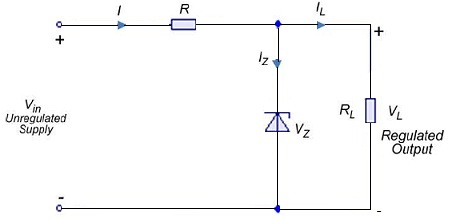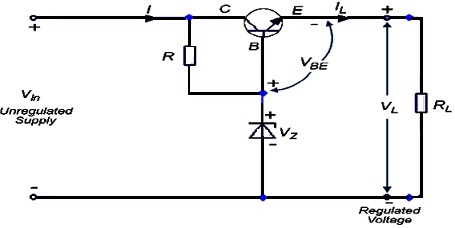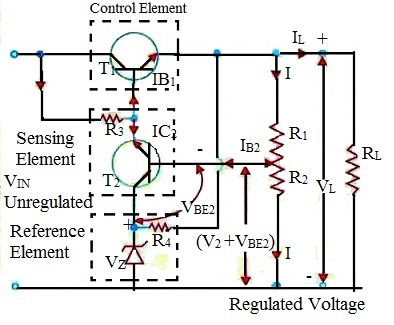#### Regulation of Output Voltage, Physics tutorial

Voltage Regulation:

In unregulated power supply, output voltage changes whenever input supply voltage or load resistance changes. It is never constant. Change in voltage from no-load to full-load condition is known as voltage regulation. Aim of voltage regulator circuit is to decrease the variations to zero or, at least, to minimum possible value. Percentage regulation or, simply, regulation of power supply is provided by:

%regulation = (Vmax - Vmin)/Vmax X 100 minimum dc output voltage.

When we say that 10V regulated dc power supply has regulation of 0.005 per cent, it signifies that dc output voltage will differ within envelope 0.005 per cent of 10V.

Now 0.005% of 10V = 0.005X10/100

= 0.0005V = 0.5mV

Therefore, output voltage will differ by ±0.25mV. In general, %regulation = (VNL - VFL)/VFL X 100

Where, VNL = no-load or open-circuit terminal voltage of supply and

VFL = full-load terminal voltage of supply

In the ideal or perfectly regulated dc power supply, percentage voltage regulation is zero. This voltage regulation is also known as load regulation.

Zener Diode Shunt Regulator:

The simple shunt voltage regulating system utilizing Zener diode is shown below. Input voltage Vin, in fact, is unregulated output of the rectifier. This simple regulator limits output voltage variations within sensible limits around Vz in face of changing load current or changing input voltage. Obviously, Zener diode will regulate so long as it is kept in reverse conduction.Transistor Series Voltage Regulator:

It is also known as emitter-follower regulator as voltage at emitter follows base voltage. In this set-up, transistor behaves like the variable resistor whose resistance is found out by base current. It is known as pass transistor as total current to be regulated passes through it.Remembering polarities of different voltages, they are associated by equation derived from KVL

VL + VBE - VZ = 0

Therefore VBE = VZ - VL

When current demand is increased by decreasing RL, VL, tends to decrease. It will increase VBE as VZ is fixed. This will increase forward bias of transistor thereby increasing its level of conduction. This, is turn, will lead to decrease in collector- emitter resistance of transistor that will slightly increase input current to compensate for decrease in RL so that VL = (ILRR)will remain at constant value. Incidentally, R is utilized for restricting current passing through Zener diode.

Controlled Transistor Series Regulator:

Circuit using second transistor T2 as sensing element is shown. It has extra feature of control with help of potentiometer R1 - R2. It will be assumed that I is much greater than IB2. Now, there is drop of VL on (R1R2) and drop of (VZ VBE2) across R2.VL/(VZ + VBE2) = (R1 + R2)/R2 or VL = ((R1 + R2)/R2)(VZ + VBE2)

If potentiometer is adjusted so that R2 decreases, then VL increases and vice versa. Assume RL is decreased, then, IL increases but VL decreases. Decrease in VL decreases IB2 and IC2. Assuming I3 to be relatively constant (or decreasing only slightly), IB1 is increased thereby decreasing terminal (collector-emitter) resistance of T1. This leads to decrease in VCE1 thereby offsetting decrease in VL that is, thus, returned to original value. In sequential logic, we have

VL↓ IB2↓ IC2↓ IB1↑ VCE1↓ V2

Transistor Shunt Voltage Regulator:

It uses transistor in shunt configuration.

As path AB is in parallel across VL, we have from Kirchhoff's Voltage Law

VL - VZ - VBE = 0 or VBE = VL - VZ (fixed)

Since VZ is fixed, any decrease or increase in VL will have corresponding effect on VBE. Assume, VL decreases, then as seen from relation, VBE also decreases. Consequently, IB decreases, therefore, IC(=βIB) decreases, thereby decreasing I and therefore VR(=IR). As a result, VL increases since at all times

Vin = VR + VL or VL = Vin - VR

In sequential logic,

Vz↓ VBE↓ IB↓ IC↓ IR↓ VR↓ VL

Same line of logic applies in case VL tries to increase.

Transistor Current Regulator:

The main function of current regulator is to maintain fixed current through load despite variations in terminal voltage. Such a circuit using Zener diode and PNP transistor is shown. Assume, because of drop in VL, current IL(=IC)is decreased. This will decrease IE(≈IC). Therefore, drop across RE i.e. VRE will decrease. As per Kirchhoff's Voltage Law

-VRE - VBE + VZ = 0 or VBE = VZ - VRE

Therefore, a decrease in VRE will increase VBE and, therefore, conductivity of transistor thereby keeping IL at fixed level. Similar logic applies when there is increase in VL.

Variable Feedback Regulator:

Regulators give non-adjustable output voltage. This would be fine if only single value of regulated voltage is needed. A feedback regulator that gives different values of regulated dc voltage. T1 is pass transistor and T2 is feedback transistor whose job is to give ample output (i.e. load) voltage. It offsets any change in output voltage. As potentiometer R3 is connected in parallel with Zener diode D, it has Zener voltage VZ applied across it. Voltage across wiper differs from 0 to Vz. Capacitor C makes sure that voltage across D and R3 doesn't change suddenly.

Voltage at base of T2 is 0.7V more positive than voltage at its emitter. Its emitter voltage and therefore base voltage can be changed with help of R3. As base of T2 is tied to output, it is liable for giving output or load voltage. Voltage VCE1 across pass transistor is given by difference of input voltage and output voltage. Current through T1 is equal to load current. R2 prevents saturation of transistors whereas R1 restricts current flowing through D.

Working of feedback transistor can be described as follows:

• As base voltage of T2 is directly related to vout it will change if Vout changes. Base and collector of T2 are 180o out of phase with each other. If base voltage increases because of increase in Vout collector voltage would decrease. Now, collector of T2 controls base of T2. As base voltage of T1 decreases, its collector-emitter resistance increases that lowers load current. This, in turn, lowers output voltage thereby offsetting attempted increases in Vout. Opposite of these steps gives the action of attempted decrease in output voltage.

Basic Op-Amp Series Regulator:

Its operations as follows:

Potentiometer R2 - R3 senses any change in out-put voltage Vout. When Vout tries to decrease due to decrease in Vin or due to increase in IL, proportional voltage decrease is applied to inverting output of op-amp by potentiometer. As, other op-amp input is held by Zener voltage at fixed reference voltage VREF, small difference voltage (known as error voltage) is developed across two inputs of op-amp. This difference voltage is amplified and op-amp's output voltage increases. This increase in voltage is applied to base of T1 causing emitter voltage (=Vout) to increase till voltage to inverting input again equals reference (Zener) voltage. This action offsets attempted decrease in output voltage therefore keeping it almost constant. Opposite action takes place if output voltage attempts to increase.

Basic Op-Amp Shunt Regulator:

Here, control element is the series resistor R1 and transistor T1 in parallel with load. In such a regulator, regulation is attained by controlling current through T1.

Working:

When output voltage attempts to decrease because of change in either input voltage or load current or temperature, attempted decrease is sensed by R3 and R4 and applied to non-inverting input of op-amp. Resulting difference in voltage decreases op-amp's output, driving T1 less therefore decreasing its collector current(shunt current), and increasing its collector-to-emitter resistance. As collector-to-emitter resistance serves as voltage divider with R1, this action offsets attempted decrease in output voltage and therefore, maintains it at constant value. Opposite action takes place when output voltage attempts to increase. Shunt regulator offers inherent short-circuit protection.

Switching Regulators:

In linear regulators considered so far, control element i.e. transistor conducts all the time, amount of conduction varying with changes in output voltage or current. Because of continuous power loss, efficiency of such a regulator is decreased to or 50% less.

The switching regulator is different as its control element operates like the switch i.e. either it is saturated (closed) or cut-off (open). Therefore, there is no needless wastage of power that results in higher efficiency of or more 90%. Switching regulators are of three kinds:

• Step-down regulator
• Step-up regulator and
• Inverting regulator

Step-Down Switching Regulator:

In this regulator, Vout is always less than Vin. Unregulated positive dc voltage is applied to collector of NPN transistor. The series of pulses from oscillator is sent to base of transistor T that gets saturated (closed) on each of positive pulses. It is so because a NPN transistor requires positive voltage pulse on its base to turn ON. The saturated transistor serves as closed switch, therefore it allows Vin to send current L through and charge C to value of output voltage during on-time (TON) of pulse. Diode D1 is reverse-biased at this point and therefore, doesn't conduct.

Ultimately when positive pulse turns to zero, T is cut-off and serves like open switch during off period (TOFF) of pulse. Collapsing magnetic field of coil produces self-induced voltage and keeps current flowing by returning energy to circuit.

Value of output voltage depends on input voltage and pulse width that is on-time of transistor. When on-time is increased relative to off- time, C charges more therefore increases Vout. When TON is decreased, C discharges more therefore decreasing Vout. By adjusting duty cycle (TON/T) of transistor, Vout can be varied.

Thus Vout = Vin(TON/T)

Where T is period of ON-OFF cycle of transistor and is related to frequency by T= 1/f.

Also, T = Ton + TOFF and ratio (TON/T) is known as duty cycle. Regulating action of circuit is as follows:

When Vout tries to decrease, on-time of transistor is increased causing an additional charge on capacitor C to offset attempted decrease. When Vout tries to increase, TON of transistor is decreased causing C to discharge enough to offset attempted increase.

Step-Up Switching Regulator:

When transistor T turns ON on arrival of positive pulse at its base, voltage across L increases rapidly to Vout - VCE(sat) and magnetic field of L expands quickly. During on-time of transistor, VL keeps decreasing from its initial maximum value. longer transistor is ON, the smaller VL becomes.

Step-up Switching Regulator

When transistor turns OFF, magnetic field of L collapses and its polarity reverses so that its voltage adds to input voltage therefore producing output voltage greater than input voltage. During off-time of transistor, D2 is forward-biased and permits C to charge. Variations in Vout because of charging and discharging action are adequately smoothed by filtering action of L and C.

It may be noted that shorter on-time of transistor, greater the inductor voltage and therefore greater the output voltage (as greater VL adds to Vin). On the other hand, longer on-time, the smaller the inductor voltage and therefore, lesser the output voltage (because smaller VL adds to Vin). Regulating action can be explained as follows:

When Vout tries to decrease (due to either increasing load or decreasing Vin), transistor on time decreases thereby offsetting tried decrease in Vout. When Vout attempts to increase, on-time increases and attempted increase in Vout is offset.

• As seen, output voltage is inversely related to duty cycle.

Therefore Vout = Vin(T/TON)

Inverting Switching Regulator

The basic diagram of such a regulator is shown. This regulator gives output voltage that is opposite in polarity to input voltage.

When transistor turns ON by positive pulse, inductor voltage VL jumps to Vin - VCE(sat) and magnetic field of inductor expands rapidly. When transistor is ON, diode D2 is reverse-biased and VL decreases from its initial maximum value.

When transistor turns OFF, magnetic field collapses and inductor's polarity reverses. This forward-biases D2, charges C and produces the negative output voltage. This repetitive ON-OFF action of transistor generates repetitive charging and discharging which is smoothed by LC filter action. As in case of step-up regulator, lesser the time for which transistor is ON, greater output voltage and vice versa.

Tutorsglobe: A way to secure high grade in your curriculum (Online Tutoring)

Expand your confidence, grow study skills and improve your grades.

Since 2009, Tutorsglobe has proactively helped millions of students to get better grades in school, college or university and score well in competitive tests with live, one-on-one online tutoring.

Using an advanced developed tutoring system providing little or no wait time, the students are connected on-demand with a tutor at www.tutorsglobe.com. Students work one-on-one, in real-time with a tutor, communicating and studying using a virtual whiteboard technology.  Scientific and mathematical notation, symbols, geometric figures, graphing and freehand drawing can be rendered quickly and easily in the advanced whiteboard.

Free to know our price and packages for online physics tutoring. Chat with us or submit request at info@tutorsglobe.com